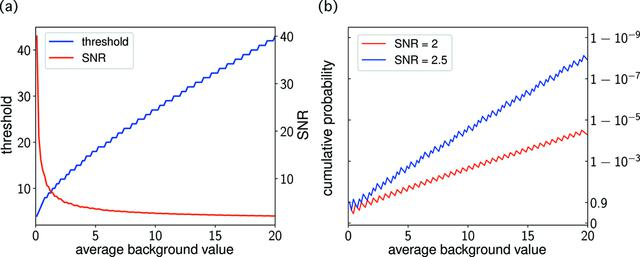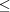disable zoom     view article Figure 1 (a) The photon count thresholds determined by equation (1)with ∊ = 10−5. The SNR is defined as the ratio of the thresholds to the background estimates. (b) The cumulative probabilities Pb(Kb × SNR) to measure a photon count K that is no larger than the thresholds b × SNR, defined by fixed values of SNR over a range of background estimates b.

IUCrJ
Volume 5| Part 5| September 2018| Pages 548-558
ISSN: 2052-2525
Follow IUCrJ